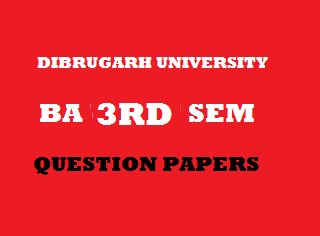## Wednesday, January 02, 20192013
(November)
ECONOMICS
(General)
Course: 301
(Public Economics)
Full Marks: 80
Pass Marks: 32
Time: 3 hours
The figures in the margin indicate full marks for the questions

1. Choose the correct option/Answer the following: 1x8=8
1. According to Dalton, social advantage will be maximum, when
1. Marginal social benefit > marginal social sacrifice.
2. Marginal social benefit < marginal social sacrifice.
3. Marginal social benefit = marginal social sacrifice.
4. Total social benefit = total social sacrifice.
1. Which one of the following forms of equal sacrifice principle is the most progressive?
1. Equal absolute sacrifice.
2. Equal marginal sacrifice.
3. Equal proportional sacrifice.
4. All are equal.
1. Ifthe burden of the tax will be upon
2. Sellers.
4. Equally between buyers and sellers.
1. Who divided the reasons of growth of public expenditure into extensive increase and intensive increase?
1. F. Shirras.
2. H. Dalton.
1. Mention one measure of repayment of public debt.
2. ____ is an item of revenue expenditure.
1. Subsidies.
2. Repayment of loans.
3. Construction of School building.
4. All of the above.
1. One source of tax revenue of the State Government is
1. Death duty.
2. Land revenue.
3. Corporate tax.
4. Income tax.
1. Write a tax levied by municipalities.
2. Write short notes on any four of the following (within 150 words each): 4x4=16
1. Objective tests for achieving the condition of maximum social advantage.
2. Adam Smith’s canons of taxation.
3. Objectives of public expenditure.
4. Limitations of fiscal policy.
5. Problem of tax evasion in India.
6. Main features of the Union Budget of 2013 – 14.
Answer the following questions (within 500 words each):
3. (a) What is public finance? Mentioning the main components of public finance, explain their importance.    2+9=11
Or
(b) Examine the role of public finance in a developing economy with special reference to India.
4. (a) Explain the effects of taxation on production and distribution. 11
Or
(b) What do you mean by taxable capacity? Discuss the factors which govern the taxable capacity of a country. What should be the limit of taxable capacity? 2+7+2=11
5. (a) Write an explanatory note on the impact of public expenditure on income distribution and economic stability. 6+6=12
Or
(b) Explain the important objectives of public borrowing. What do your understand by direct burden of debt? 9+3=12
6. (a) What is budget? What are the main objectives of budgeting? Distinguish between revenue deficit and fiscal deficit. 1+7+3=11
Or
(b) In what ways does fiscal policy help in attaining the objectives of economic growth and price stabilization? 6+5=11
7. (a) Discuss the main features of Indian tax system. Mention the taxes on which this tax system is mainly dependent upon. 8+3=11
Or
(b) Explain the principles of distribution of financial resources between the Centre and the States. 11

***